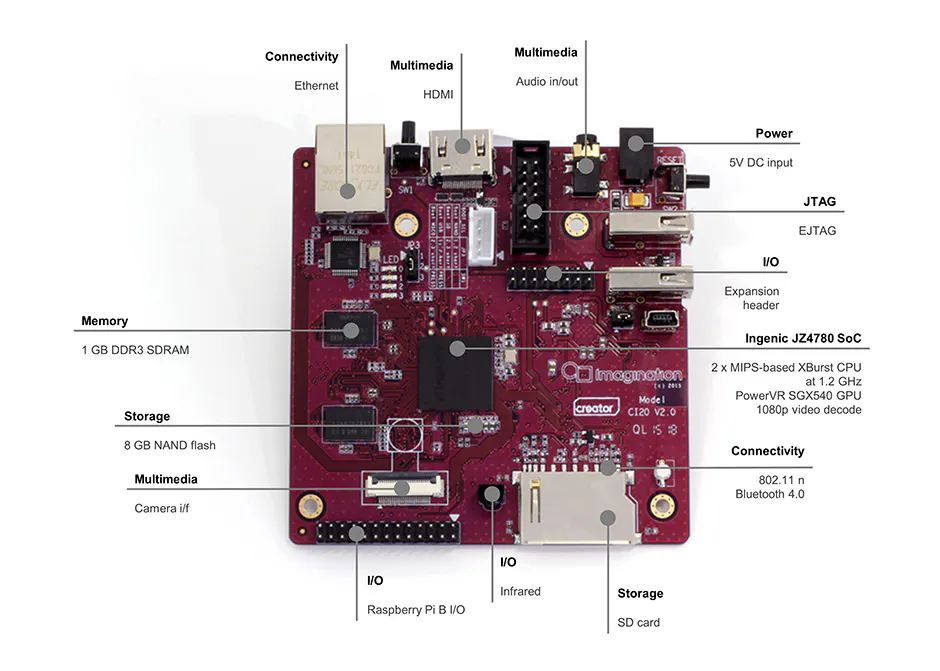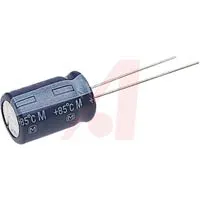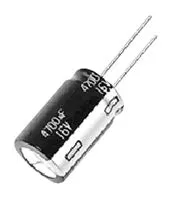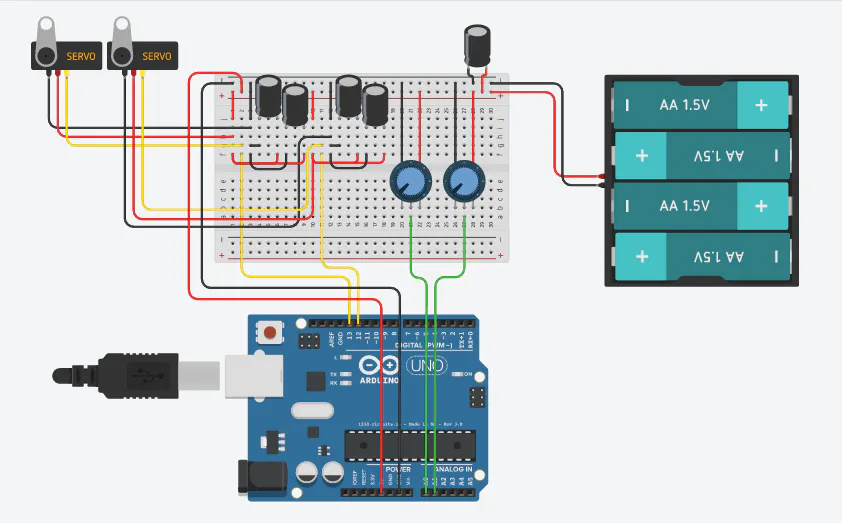Published

# Robotic Arm And 3D Modeling

A 3D printed robotic arm, controlled through an Arduino, also being 3D rendered in Python OpenGL, using the Ci20 board.

## Things used in this project

### Hardware componentsCreator Ci20
×1Arduino UNO & Genuino UNO
×1
 Servos (Tower Pro MG996R)
×2Jumper wires (generic)
×1
×1Capacitor 10 µF
×3Capacitor 1 µF
×2

### Hand tools and fabrication machines3D Printer (generic)

## Schematics

### Arduino and Ci20 Schematic

Depicted in this schematic is the circuit to control the servos and connect them to the arduino. (Not Depicted: The Arduino's Tx line is also connected to the Ci20's UART0 RX Pin, located on GPIO pin 10 of the main header. The Ci20 also supplies 3.3v to the arduino's VIN pin, and the ground pins of the arduino and Ci20 are also connected.)## Code

### Python OpenGL Robotic Arm Model

Python
A program written using the python opengl library. It features a controllable arm model and movable camera.
Key Controls:
- L and R arrows: translate x
- U and D arrows: translate y
- J and L keys: translate z

- A and D keys: x rotation
- W and S keys: y rotation
- I and K keys: z rotation

- G and H keys: Arm rotation
```import serial
import time
import sys
import math
import pygame
from pygame.locals import *

from OpenGL.GL import *
from OpenGL.GLU import *

#Initialize Serial Port (On uart0)
port = serial.Serial("/dev/ttyS0",baudrate=9600,timeout=1)

#Map function variables
in_min=0
in_max=1024
out_min=0
out_max=90

def axes():
axesVerticies=(
(0,0,0),
(50,0,0),
(0,50,0),
(0,0,50),
(50,50,0),
(0,50,50),
(50,0,50)
)
axesEdges= (
(0,1),
(0,2),
(0,3),
(1,4),
(1,6),
(2,4),
(2,5),
(3,5),
(3,6)
)
axesSurfaces= (
(0,1,6,3),
(0,1,4,2),
(0,2,5,3)
)
axesColors = (
(1,.5,.5),#red
(.5,1,.5),#green
(.5,.5,1),#blue
(1,.5,.5),
(.5,1,.5),
(.5,.5,1),
(1,.5,.5),
(.5,1,.5),
(.5,.5,1),
(1,.5,.5),
(.5,1,.5),
(.5,.5,1)
)

i=0
for surface in axesSurfaces:
i+=1
glColor3fv(axesColors[i])
#Color the different surfaces red, blue, and green
for vertex in surface:
glVertex3fv(axesVerticies[vertex])

glEnd()

#Build Axes
glBegin(GL_LINES)
for edge in axesEdges:
for vertex in edge:
glVertex3fv(axesVerticies[vertex])
glEnd()

(0,0,0),
(0,3,0),
(20,0,0),
(20,5,0),
(0,0,.5),
(0,3,.5),
(20,0,.5),
(20,5,.5)
)
(0,1),
(0,2),
(0,4),
(1,3),
(1,5),
(2,3),
(2,6),
(3,7),
(4,5),
(4,6),
(5,7),
(6,7)
)
(0,1,2,3),
(4,5,6,7),
(0,1,4,5),
(2,3,6,7),
(0,2,4,6),
(1,3,5,7)
)

glRotatef(90,1,0,0)
glTranslatef(25,25,25)#Move to center

glRotate(-a,0,0,-1)#Rotate Radius by a, a is changed on keypress
glTranslatef(-20,0,0)#Keep the Radius the same distance away after rotation

glEnd()

glBegin(GL_LINES)
for vertex in edge:
glEnd()

def humerus():
humerusVerticies= (
(0,0,0),
(0,5,0),
(25,-1,0),
(25,6,0),
(0,0,.5),
(0,5,.5),
(25,-1,.5),
(25,6,.5)
)
humerusEdges= (
(0,1),
(0,2),
(0,4),
(1,3),
(1,5),
(2,3),
(2,6),
(3,7),
(4,5),
(4,6),
(5,7),
(6,7)
)
humerusSurfaces= (
(0,1,2,3),
(4,5,6,7),
(0,1,4,5),
(2,3,6,7),
(0,2,4,6),
(1,3,5,7)
)

#Position the Humerus Vertically
glRotatef(90,0,0,1)
glRotatef(90,1,0,0)
glTranslatef(25,25,25)

for surface in humerusSurfaces:
glColor3fv((1,1,1))#Color the Humerus White

glEnd()

#Build the Humerus
glBegin(GL_LINES)

for edge in humerusEdges:
for vertex in edge:
glVertex3fv(humerusVerticies[vertex])
glEnd()

def map(rcv,in_min,in_max,out_min,out_max):
#This function is from the arduino. It maps the values
#of one input, onto a different range, creating the output
return(rcv-in_min*(out_max-out_min)/(in_max-in_min)+out_min)

def serialInput():
#This function reads the serial port, uart0
#It then processes the data into a usable variable

#This Function is broken and does not work currently
int(rcv)
a = map(rcv,in_min,in_max,out_min,out_max)
return(a)

def text(x,y,z):
#Print out the position of the camera
#Rotation isn't implemented, so it won't be accurate
print("Position:",x,y,z)
sys.stdout.write("\033[F")

def main():

#Build Pygame Window
pygame.init()
display = (800,800)
pygame.display.set_mode(display, DOUBLEBUF|OPENGL)

gluPerspective(45, (display/display), 0.1, 300.0)

#Set Camera Position
glRotatef(-45,-1,0,0)#Rotate Camera down
glRotatef(45,0,-1,0)#Rotate Camera turn
glTranslatef(-100,-150,-100)#Translate the camera to the corner

#I had problems figuring out how to properly arrange these functions
#There order will effect their outcomes; Changing one value can
#have drastically different results.
#Rotating the camera first is important, because if you
#translate it first, the rotation after it is then multiplied
#with the translation, causing it to do things you wouldn't
#expect.

#Variables

a=0

#Coordinates
x=0
y=0
z=0

#Keystates
KLEFT=False
KRIGHT=False
KUP=False
KDOWN=False
KJ=False
KL=False
KA=False
KD=False
KW=False
KS=False
KI=False
KK=False
KG=False
KH=False

#Infinite while loop
while True:
#Event Handling loop
for event in pygame.event.get():
if event.type == pygame.QUIT:
pygame.quit()
quit()
#Kill Window

#KEYEVENT KEYDOWN HANDLING
if event.type == pygame.KEYDOWN:
#TRANSLATE
if event.key == pygame.K_LEFT:
KLEFT=True
if event.key == pygame.K_RIGHT:
KRIGHT=True
if event.key == pygame.K_UP:
KUP=True
if event.key == pygame.K_DOWN:
KDOWN=True
if event.key == pygame.K_j:
KJ=True
if event.key == pygame.K_l:
KL=True

#ROTATE
if event.key == pygame.K_a:
KA=True
if event.key == pygame.K_d:
KD=True
if event.key == pygame.K_w:
KW=True
if event.key == pygame.K_s:
KS=True
if event.key == pygame.K_i:
KI=True
if event.key == pygame.K_k:
KK=True
#Arm Control
#Optional key usage to move arm model

if event.key == pygame.K_g:
KG=True
if event.key == pygame.K_h:
KH=True

#KEYEVENT KEYUP HANDLING
if event.type == pygame.KEYUP:
#TRANSLATE
if event.key == pygame.K_LEFT:
KLEFT=False
if event.key == pygame.K_RIGHT:
KRIGHT=False
if event.key == pygame.K_UP:
KUP=False
if event.key == pygame.K_DOWN:
KDOWN=False
if event.key == pygame.K_j:
KJ=False
if event.key == pygame.K_l:
KL=False

#ROTATE
if event.key == pygame.K_a:
KA=False
if event.key == pygame.K_d:
KD=False
if event.key == pygame.K_w:
KW=False
if event.key == pygame.K_s:
KS=False
if event.key == pygame.K_i:
KI=False
if event.key == pygame.K_k:
KK=False

#Arm Angle
#Optional key usage to move arm model

if event.key == pygame.K_g:
KG=False
if event.key == pygame.K_h:
KH=False

#KEY PRESS ACTIONS

#TRANSLATE
if KLEFT==True:
glTranslatef(5,0,0)
x+=5
if KRIGHT==True:
glTranslatef(-5,0,0)
x-=5
if KUP==True:
glTranslatef(0,-5,0)
y+=5
if KDOWN==True:
glTranslatef(0,5,0)
y-=5
if KJ==True:
glTranslatef(0,0,-5)
z+=5
if KL==True:
glTranslatef(0,0,5)
z-=5

#ROTATE
if KA==True:
glRotate(5,0,1,0)
if KD==True:
glRotate(5,0,-1,0)
if KW==True:
glRotate(5,-1,0,0)
if KS==True:
glRotate(5,1,0,0)
if KI==True:
glRotate(5,0,0,-1)
if KK==True:
glRotate(5,0,0,1)

#Arm Angle
#Optional key usage to move arm model
if KG==True:
a+=5

if KH==True:
a-=5

#Another area I had trouble with was understanding how the camera
#translation, and rotation, all worked together. An assumption
#was that when translate or rotate was called, the camera
#was doing the moving. This caused me major confusion, due
#to me then not understanding how to translate and rotate
#objects.

#This is an important distinction:
#	-The world is not static and the camera moves
#	-But the camera is static and the world moves
#Once I realized the second statement is true, then everything
#became much clearer, and I could continue working on my project
#with much less confusion.

glClear(GL_COLOR_BUFFER_BIT|GL_DEPTH_BUFFER_BIT)

#Get joystick input
#serialInput()

#Draw shapes
axes()

glPushMatrix()
glPopMatrix()

glPushMatrix()
humerus()
glPopMatrix()

text(x,y,z)

pygame.display.flip()
pygame.time.wait(10)

main()
```

### Python Serial Test

Python
This was a test program for testing out the serial port of the Ci20.
```import serial
port = serial.Serial("/dev/bus/usb/003",baudrate=9600,timeout=3.0)
#"/dev/bus/usb/003"
while True:
print(rcv)
```

### Arduino Joystick to Servo Control

Arduino
This program takes the input of the joysticks and maps the servos position to it.
```#include <Servo.h>

Servo servo1;
Servo servo2;

void setup() {

Serial.begin(9600);
while(!Serial){
//Wait until Serial port opens before sending data
}
pinMode(A0,INPUT);
pinMode(A1,INPUT);
pinMode(10,OUTPUT);
pinMode(9,OUTPUT);
servo1.attach(10);
servo2.attach(9);
}

void loop() {

servoFunction();

}

void servoFunction(){

//Send Joystick Position to the serial port
Serial.println(x);
Serial.println(' ');
Serial.println(y);

//Map motor position to joystick position
int s1position = map(x, 0, 1023, 500, 2300);
int s2position = map(y, 1023, 0, 600, 2400);
//Range of servo1: 500-2300
//Range of servo2: 600-2400
//Each Servo has a specific range for its end stops
//This was determined through trial and error

//Set motor position to joystick position
servo1.writeMicroseconds(s1position);
servo2.writeMicroseconds(s2position);

}
```

## Credits

### Samuel Jasmer

1 project • 3 followers
Mechanical Engineer, college freshman
Thanks to Sentdex.